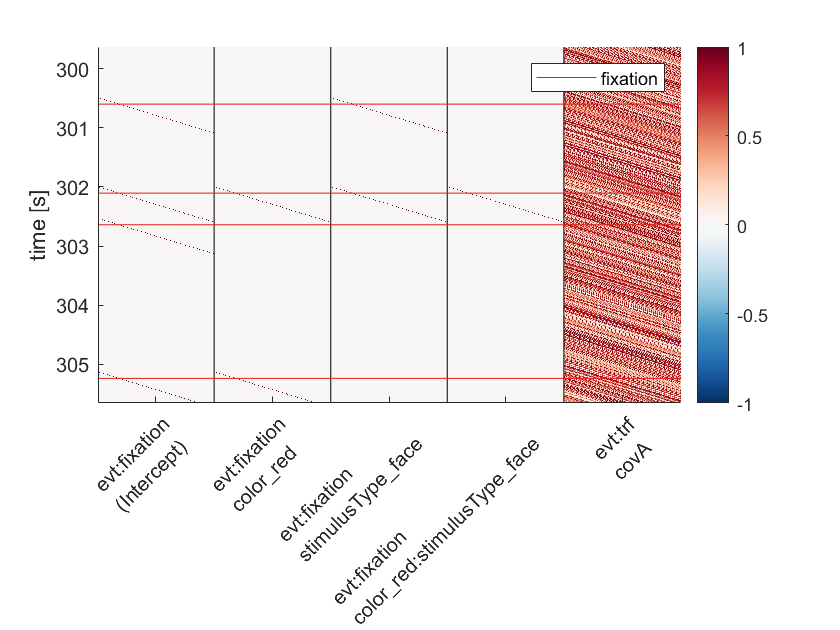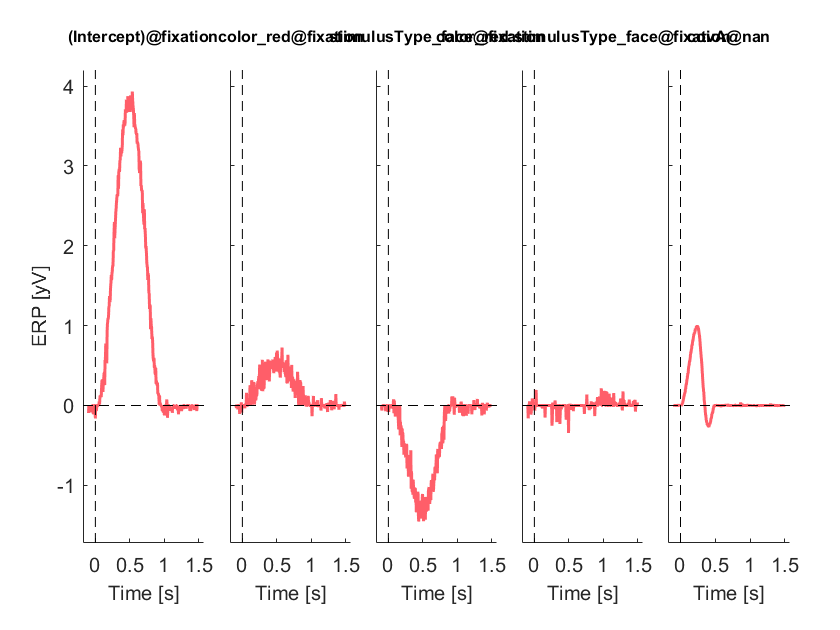init_unfold
Starting unfold toolbox. Adding subfolders and other toolboxes to path... Done.

# Introduction to TRF

In this tutorial we will introduce the difference between rERP-style predictors (e.g. using Stick-Functions/FIR-deconvolution) and TRF (temporal response functions, with predictors that are the size of the continuous EEG, e.g. an auditory envelope). Note that this distinction is not often made in the literature, but we think it is conceptually important and pragmatic to differentiate these two, even though mathematically they are very related.
In this tutorial we will do the following:
• Generate a designmatrix for TRFs and estimate them
• Combine rERPs and TRFs in one fit
% We will first simulate data based on the car/face, green/red design.
EEG = tutorial_simulate_data('trf');
eeg_checkset note: upper time limit (xmax) adjusted so (xmax-xmin)*srate+1 = number of frames
size(EEG.data)
As you can see, EEG.data has two channels now. But only the first one contains actual EEG data, the second one contains a covariate that spans the recording session. In fMRI this is usually some kind of motion regressor, in EEG this is often a feature of an auditory signal. But this could be position in space, eye movement position, optical flow, etc.. The underlying idea is that the brain responds continously to the changing input. The brain acts as a filter and consequently we can recover the "filter-kernel" (note that an ERP can be seen as such a filter-kernel as well, but to a single impulse-response).
Now we gonna add the channel as a predictor to our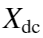designmatrix and timeexpand it.
Warning: EXPERIMENTAL FUNCTION - RISKY USAGE
Could not find Xdc, assuming only TRFs are fitted and initializing the Xdc field Important: If you want to combine rERPs and TRFs you HAVE to use uf_timeexpand BEFORE uf_timeexpandDesignmat_addTRF
Visualizing the designmatrix clearly shows the timeshifted pattern.
uf_plotDesignmat(EEG_trf,'timeexpand',1)
ylim([300 301])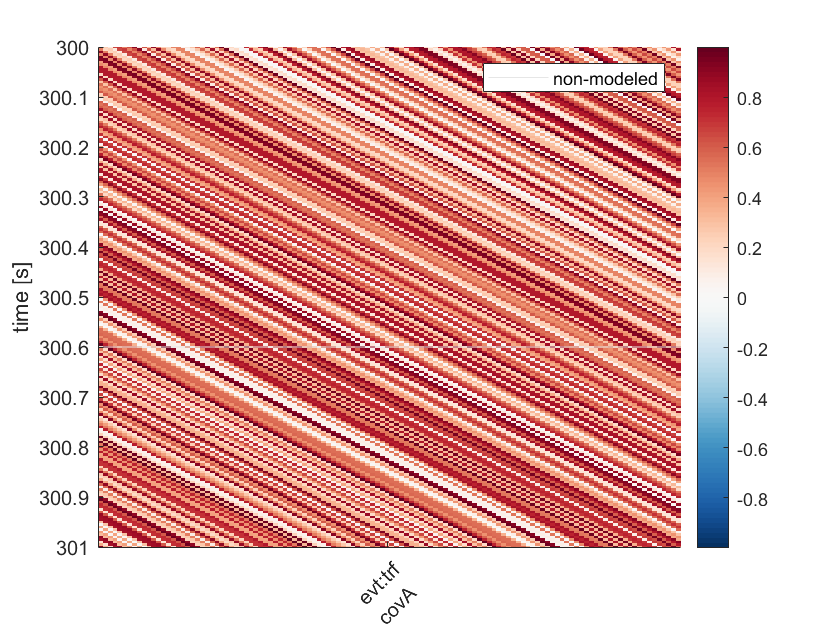It might also become very obvious, that such a TRF timeshift may introduce much more collinearity than the normal rERP approach. Therefore it is in most cases mandatory to use some kind of regularization (this is not strictily necessary in this tutorial, as our predictor was generated without any autocorrelation over time, thus each timelag is independent from eachother. Still, we are going to use regularisation because in most TRF-cases it is the right thing to do)
Note: If you have many predictors, this will make the designmatrix quite unspare. Most likely you will need more RAM for your analysis.
EEG_trf= uf_glmfit(EEG_trf,'method','glmnet','channel',1); %Glmnet uses L2 norm by default
uf_glmfit(): Fitting deconvolution model...solving the equation system solving electrode 1 (of 1 electrodes in total)... took 18.3s LMfit finished
ufresult = uf_condense(EEG_trf)
ufresult = struct with fields:
unfold: [1×1 struct] beta: [1×120 double] times: [1×120 double] chanlocs: [] param: [1×1 struct]
uf_plotParam(ufresult)
a single channel detected, none specified, thus using this one plotting all parameters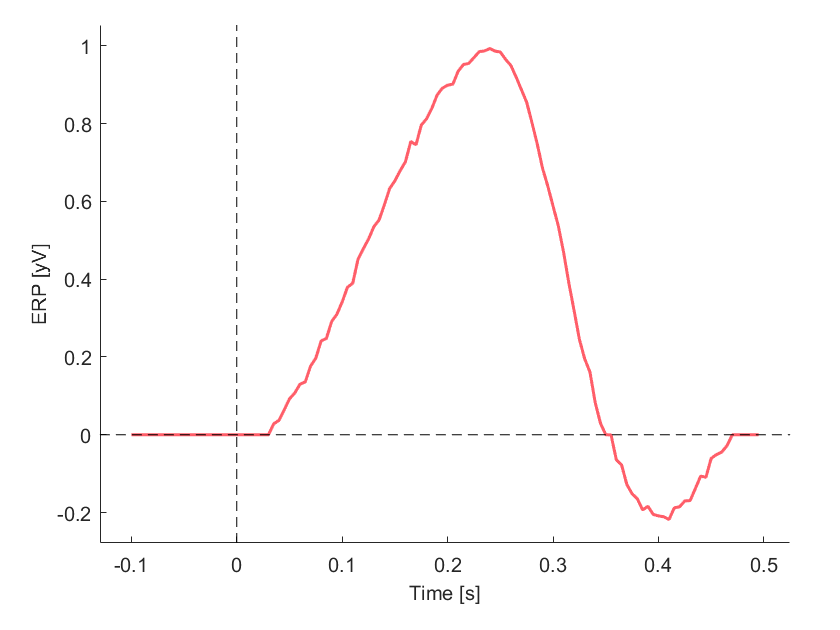We successfully recovered the TRF filter-kernel.

# Combining rERPs and TRFs

We now combine a rERP and a TRF model in one go.
First define the rERP design (identical to tutorial_2x2)
EEG = uf_designmat(EEG,'eventtypes','fixation','formula','y~cat(stimulusType)*cat(color)');
Modeling 413 event(s) of [fixation] using formula: y~stimulusType*color
EEG = uf_timeexpandDesignmat(EEG,'timelimits',[-0.1,0.5]);
uf_timeexpandDesignmat(): Timeexpanding the designmatrix... ...done